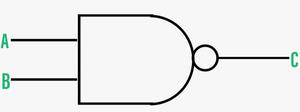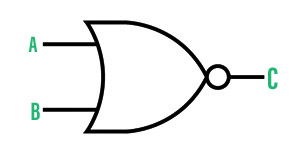Open in App
Not now

# Difference Between NAND GATE and NOR GATE

• Difficulty Level : Basic
• Last Updated : 12 Sep, 2022

NAND and NOR gates are universal logic gates, which means any Boolean expression can be implemented without using any other gate. Using only NOR gates or only NAND gates all other Boolean expressions can be created unlike other gates. In this article, we will discuss the difference between NAND GATE and NOR GATE.

### NAND GATE:

A NAND gate, sometimes known as a ‘NOT-AND’ (Negated Gate) gate, is essentially a Not gate followed by an AND gate. NAND Gate is one of the Universal Gates.

• This gate’s output is 1 only if none of the inputs is 1. Alternatively, when all of the inputs are not high and at least one is low, the output is high.
• If there are two inputs A and B, the Boolean expression for the NAND gate is Y=(A.B)’
• The output is the exact inverse of the AND gate for all possible input combinations in the NAND gate.
• NAND gate follows the commutative law as (AB)’ = (BA).’

Here, A and B are the inputs and Y is the output. NAND logic gate can be achieved by multiplying all the inputs and then complementing the multiplied result.

Symbol:NAND Gate

Truth Table of NOT Gate:

Truth Table Of NAND Gate:

For n=2 (where n is the number of inputs).

Combining the output of AND with NOT results in NAND Gate output.

Applications of NAND Gate:

1. NAND gates help detect if a single input to a digital system has gone low.
2. Used in Alarm Circuit.

### NOR GATE:

The NOR gate (negated OR) gives an output of 1 if both inputs are 0. NOR Gate is one of the Universal Gates.

A NOR gate, sometimes known as a “NOT-OR” gate, consists of an OR gate followed by a NOT gate.

• This gate’s output is 1 only when all of its inputs are 0. Alternatively, when all of the inputs are low, the output is high.
• The Boolean statement for the NOR gate is Y=(A+B)’ if there are two inputs A and B.
• The NOR gate is also called the active LOW AND gate.

Here, A and B are the inputs and Y is the output. NOR logic gate can be achieved by the addition of all the inputs and then complementing the added result.

Symbol:NOR Gate

Truth Table Of NOR Gate:

For n=2 (where n is the number of inputs).

Applications of NOR Gate:

1. Used in Combinational Circuit Example – Multipliers, Multiplexers.
2. Used in Sequential Circuit.

### Difference between NAND Gate and NOR Gate:

My Personal Notes arrow_drop_up
Related Articles